Ch 4. The Mean Value Theorem Multimedia Engineering Math Maximum & Minimum Rolle'sTheorem Mean ValueTheorem MonotonicFunctions First DerivativeTest Concavity &Inflection SecondDerivative Test
 Chapter 1. Limits 2. Derivatives I 3. Derivatives II 4. Mean Value 5. Curve Sketching 6. Integrals 7. Inverse Functions 8. Integration Tech. 9. Integrate App. 10. Parametric Eqs. 11. Polar Coord. 12. Series Appendix Basic Math Units Search eBooks Dynamics Fluids Math Mechanics Statics Thermodynamics Author(s): Hengzhong Wen Chean Chin Ngo Meirong Huang Kurt Gramoll ©Kurt GramollMATHEMATICS - CASE STUDY SOLUTION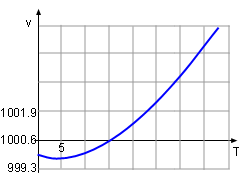Volume Between 0oC and 40oC, the volume, v, of a 1 kg solution was determined to follow the relationship       v = 999.87 - 0.06426T + 0.0085T2 - 0.00006T3 where T is the temperature. At what temperature is the mass density a maximum? Let the density, volume and the mass of the solution be ρ, v and m respectively.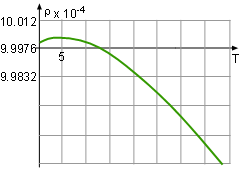Density The density is defined as ρ = mass/volume = m/v = 1000/v. In order to find the maximum dencisty in the domain between 0oC and 40oC, the following steps need to be taken: Find the critical points of the function and calculate the value at critical points. Find the value of f(0) and f(40). Compare the result of the above steps. The maximum density is the largest one.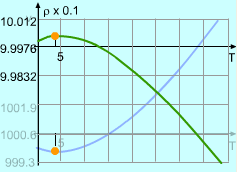Critical Point for Density and Volume are the Same The critical points is the point where the derivative of the density is 0 or does not exist. Therefore, the derivative of the density, ρ, with respect to temperature, T, is needed,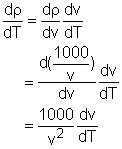Since v is not 0, the critical point of density is the same as the volume and it is easy to calculate the critical point of the volume. The formula of the volume is given      v = 999.87 - 0.06426T + 0.0085T2 - 0.00006T3 Differentiate both side of the formula with respect to temperaure gives:      dv/dT = - 0.06426 + 0.0170T - 0.00018T2 Find the temperature when dv/dT = 0.      - 0.06426 + 0.0170T - 0.00018T2 = 0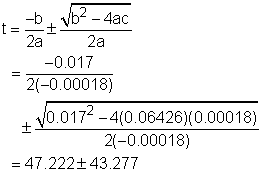So T = 90.5 or T = 3.945 It is given that the range of temperature in the volume function is from 0oC to 40oC, so the 90.5 is discarded and thus 3.945 is the solution. At this temperature, the volume of the solution is      v(3.945) = 999.87 - 0.06426T + 0.0085T2                      - 0.00006T3                   = 999.87 - 0.06426(3.945)                      + 0.0085(3.945)2 - 0.00006(3.945)3                   = 999.74 The density at temperature 3.945 is       ρ(3.945) = 1000/v = 1000/999.74 = 1.0003 With the same method, the density at the temperature of 0 and 40 can be calculated.      ρ(0) = 1.0001      ρ(3.945) = 0.993 Comparing the three density, the maximum value is 1.0003 kg/m3 at a temperature of 3.945oC.